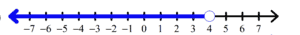In this video, we will be learning how to solve inequalities using multiplication or division. After you finish this lesson, view all of our Algebra 1 lessons and practice problems.

## Example of Solving an Inequality Using Multiplication/Division$-3x\leq-15$$\frac{-3x}{-3}\leq\frac{-15}{-3}\leftarrow$ Divide by -3 on both sides (Don’t forget to flip the inequality sign since we are dividing by a negative!)$x\geq5\leftarrow$ Graph the inequality### Example 1$8a\geq32$

To solve for$a$, we have to divide both sides by$8$$\dfrac{8a}{8}\geq{32}{8}$$b\geq4$

Then, graph the inqequality### Example 2$\dfrac{a}{2}\textless 2$

To solve for$x$, let’s multiply both sides by$2$$(2)(\dfrac{a}{2})\textless (2)(2)$$a\textless4$

Then, graph the inqequality## Video-Lesson Transcript

Let’s go over how to solve inequalities using multiplication or division

The rules are very similar to solving regular equations except for one rule.

If we multiply or divide by a negative number, we have to flip inequality.

Here are some examples:$3x \leq 12$

To solve for$x$, we have to divide both sides by$3$.$\dfrac{3x}{3} \leq \dfrac{12}{3}$$x \leq 4$

Next we have$-4x \leq 20$

Let’s solve$x$ by dividing both sides by$-4$$\dfrac{-4x}{-4} \leq \dfrac{20}{-4}$

Since we divide it by a negative number, the inequality sign should be flipped.$x \geq -5$

Let’s look at another example.$12x > -36$

So, we’ll solve$x$ by dividing both sides by$12$.$\dfrac{12x}{12} > \dfrac{-36}{12}$

Here, its negative divided by positive.

Remember the rule? If we multiply or divide by a negative number, we have to flip inequality.

In this example, we are dividing by positive$12$. So there’s no need to flip the sign.$x > -3$

Next, let’s have$\dfrac{x}{-5} \geq 40$

To solve for$x$, let’s divide both sides by$-5$.$-5 \times \dfrac{x}{-5} \geq 40 \times{-5}$

Here, we multiplied by a negative number so we have to flip the inequality sign.$x \leq -200$So in solving inequalities using multiplication or division, follow the same rules to solve for$x$.